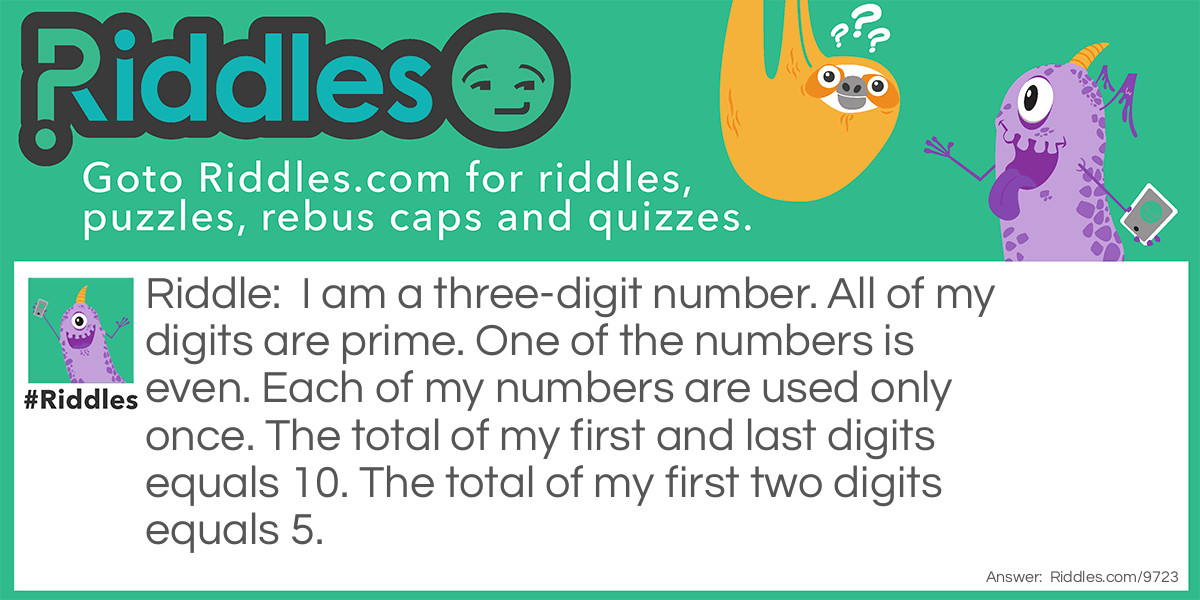ADVERTISEMENT
Riddle:

I am a three-digit number. All of my digits are prime. One of the numbers is even. Each of my numbers are used only once. The total of my first and last digits equals 10. The total of my first two digits equals 5.

Answer:

This one is fairly easy if you use elimination if you follow all the first 5 steps you get three options: 525, 327, and 723 but if you followed the last step you would reach your answer. The answer was 327.

VOTE
SHARE
COMMENTThe king of primes Riddle Meme with riddle and answer link.
ADVERTISEMENT